# 1.1 Functions and function notation  (Page 10/21)

 Page 10 / 21

Why does the horizontal line test tell us whether the graph of a function is one-to-one?

When a horizontal line intersects the graph of a function more than once, that indicates that for that output there is more than one input. A function is one-to-one if each output corresponds to only one input.

## Algebraic

For the following exercises, determine whether the relation represents a function.

$\left\{\left(a,b\right),\left(b,c\right),\left(c,c\right)\right\}$

function

For the following exercises, determine whether the relation represents $\text{\hspace{0.17em}}y\text{\hspace{0.17em}}$ as a function of $\text{\hspace{0.17em}}x.\text{\hspace{0.17em}}$

$5x+2y=10$

$y={x}^{2}$

function

$x={y}^{2}$

$3{x}^{2}+y=14$

function

$2x+{y}^{2}=6$

$y=-2{x}^{2}+40x$

function

$y=\frac{1}{x}$

$x=\frac{3y+5}{7y-1}$

function

$x=\sqrt{1-{y}^{2}}$

$y=\frac{3x+5}{7x-1}$

function

${x}^{2}+{y}^{2}=9$

$2xy=1$

function

$x={y}^{3}$

$y={x}^{3}$

function

$y=\sqrt{1-{x}^{2}}$

$x=±\sqrt{1-y}$

function

$y=±\sqrt{1-x}$

${y}^{2}={x}^{2}$

not a function

${y}^{3}={x}^{2}$

For the following exercises, evaluate the function $\text{\hspace{0.17em}}f\text{\hspace{0.17em}}$ at the indicated values

$f\left(x\right)=2x-5$

$\begin{array}{cccc}f\left(-3\right)=-11;& f\left(2\right)=-1;& f\left(-a\right)=-2a-5;& -f\left(a\right)=-2a+5;\text{\hspace{0.17em}}\text{\hspace{0.17em}}\text{\hspace{0.17em}}\text{\hspace{0.17em}}f\left(a+h\right)=2a+2h-5\end{array}$

$f\left(x\right)=-5{x}^{2}+2x-1$

$f\left(x\right)=\sqrt{2-x}+5$

$\begin{array}{cccc}f\left(-3\right)=\sqrt{5}+5;& f\left(2\right)=5;& f\left(-a\right)=\sqrt{2+a}+5;& -f\left(a\right)=-\sqrt{2-a}-5;\text{\hspace{0.17em}}\text{\hspace{0.17em}}\text{\hspace{0.17em}}\text{\hspace{0.17em}}\text{\hspace{0.17em}}f\left(a+h\right)=\end{array}$ $\sqrt{2-a-h}+5$

$f\left(x\right)=\frac{6x-1}{5x+2}$

$f\left(x\right)=|x-1|-|x+1|$

Given the function $\text{\hspace{0.17em}}g\left(x\right)=5-{x}^{2},\text{\hspace{0.17em}}$ evaluate $\text{\hspace{0.17em}}\frac{g\left(x+h\right)-g\left(x\right)}{h},\text{\hspace{0.17em}}h\ne 0.$

Given the function $\text{\hspace{0.17em}}g\left(x\right)={x}^{2}+2x,\text{\hspace{0.17em}}$ evaluate $\text{\hspace{0.17em}}\frac{g\left(x\right)-g\left(a\right)}{x-a},\text{\hspace{0.17em}}x\ne a.$

$\frac{g\left(x\right)-g\left(a\right)}{x-a}=x+a+2,\text{\hspace{0.17em}}x\ne a$

Given the function $\text{\hspace{0.17em}}k\left(t\right)=2t-1:$

1. Evaluate $\text{\hspace{0.17em}}k\left(2\right).$
2. Solve $\text{\hspace{0.17em}}k\left(t\right)=7.$

Given the function $\text{\hspace{0.17em}}f\left(x\right)=8-3x:$

1. Evaluate $\text{\hspace{0.17em}}f\left(-2\right).$
2. Solve $\text{\hspace{0.17em}}f\left(x\right)=-1.$

a. $\text{\hspace{0.17em}}f\left(-2\right)=14;\text{\hspace{0.17em}}$ b. $\text{\hspace{0.17em}}x=3$

Given the function $\text{\hspace{0.17em}}p\left(c\right)={c}^{2}+c:$

1. Evaluate $\text{\hspace{0.17em}}p\left(-3\right).$
2. Solve $\text{\hspace{0.17em}}p\left(c\right)=2.$

Given the function $\text{\hspace{0.17em}}f\left(x\right)={x}^{2}-3x:$

1. Evaluate $\text{\hspace{0.17em}}f\left(5\right).$
2. Solve $\text{\hspace{0.17em}}f\left(x\right)=4.$

a. $\text{\hspace{0.17em}}f\left(5\right)=10;\text{\hspace{0.17em}}$ b. or

Given the function $\text{\hspace{0.17em}}f\left(x\right)=\sqrt{x+2}:$

1. Evaluate $\text{\hspace{0.17em}}f\left(7\right).$
2. Solve $\text{\hspace{0.17em}}f\left(x\right)=4.$

Consider the relationship $\text{\hspace{0.17em}}3r+2t=18.$

1. Write the relationship as a function $\text{\hspace{0.17em}}r=f\left(t\right).$
2. Evaluate $\text{\hspace{0.17em}}f\left(-3\right).$
3. Solve $\text{\hspace{0.17em}}f\left(t\right)=2.$

a. $\text{\hspace{0.17em}}f\left(t\right)=6-\frac{2}{3}t;\text{\hspace{0.17em}}$ b. $\text{\hspace{0.17em}}f\left(-3\right)=8;\text{\hspace{0.17em}}$ c. $\text{\hspace{0.17em}}t=6\text{\hspace{0.17em}}$

## Graphical

For the following exercises, use the vertical line test to determine which graphs show relations that are functions.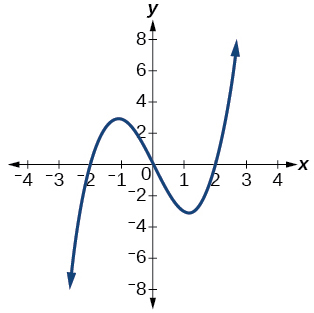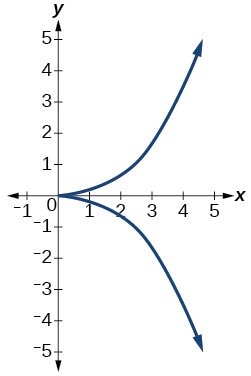not a function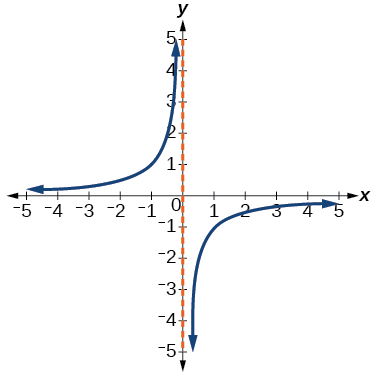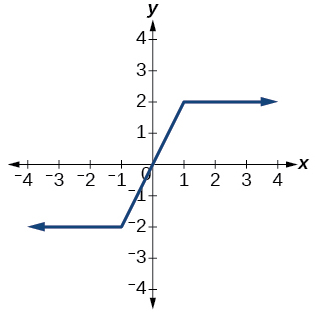function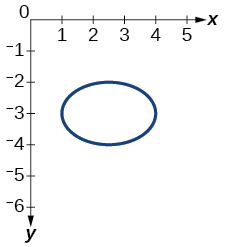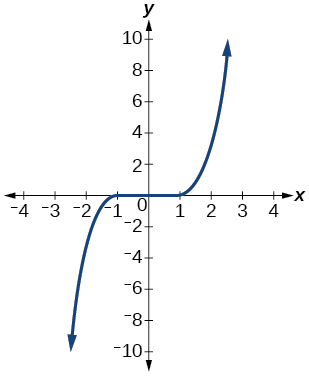function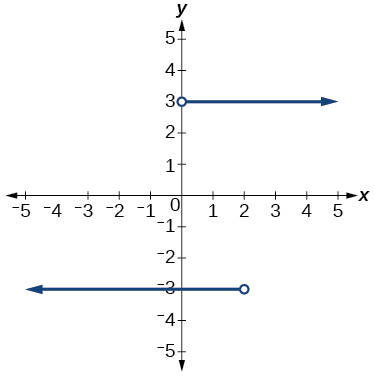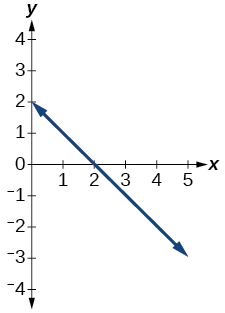function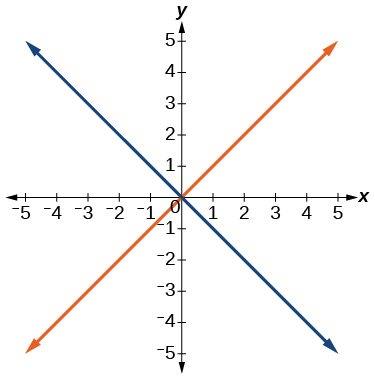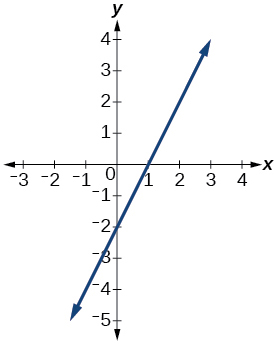function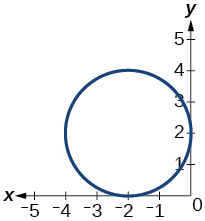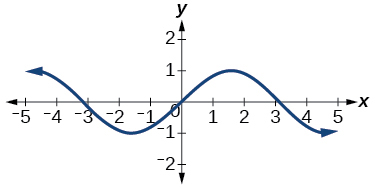function

Given the following graph,

• Evaluate $\text{\hspace{0.17em}}f\left(-1\right).$
• Solve for $\text{\hspace{0.17em}}f\left(x\right)=3.$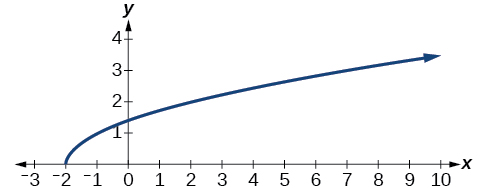Given the following graph,

• Evaluate $\text{\hspace{0.17em}}f\left(0\right).$
• Solve for $\text{\hspace{0.17em}}f\left(x\right)=-3.$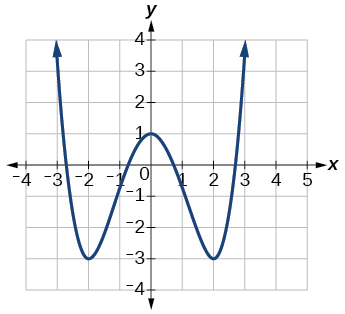a. $\text{\hspace{0.17em}}f\left(0\right)=1;\text{\hspace{0.17em}}$ b. or

Given the following graph,

• Evaluate $\text{\hspace{0.17em}}f\left(4\right).$
• Solve for $\text{\hspace{0.17em}}f\left(x\right)=1.$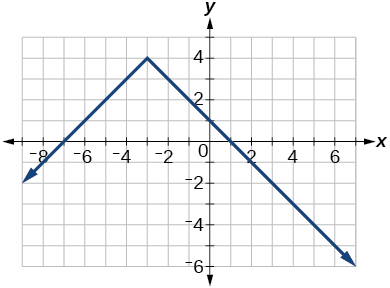For the following exercises, determine if the given graph is a one-to-one function.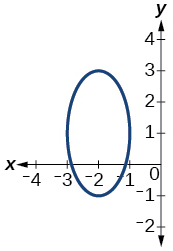not a function so it is also not a one-to-one function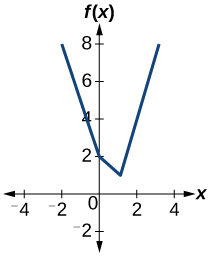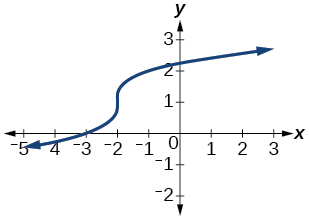one-to- one function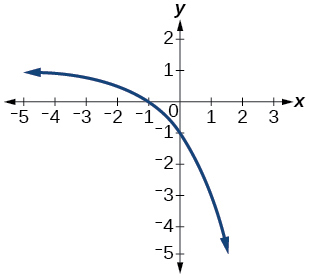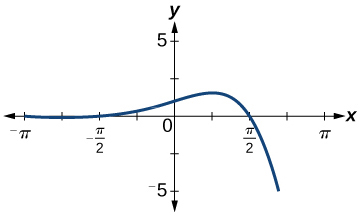function, but not one-to-one

## Numeric

For the following exercises, determine whether the relation represents a function.

$\left\{\left(-1,-1\right),\left(-2,-2\right),\left(-3,-3\right)\right\}$

$\left\{\left(3,4\right),\left(4,5\right),\left(5,6\right)\right\}$

function

$\left\{\left(2,5\right),\left(7,11\right),\left(15,8\right),\left(7,9\right)\right\}$

For the following exercises, determine if the relation represented in table form represents $\text{\hspace{0.17em}}y\text{\hspace{0.17em}}$ as a function of $\text{\hspace{0.17em}}x.$

 $x$ 5 10 15 $y$ 3 8 14

function

 $x$ 5 10 15 $y$ 3 8 8
 $x$ 5 10 10 $y$ 3 8 14

not a function

For the following exercises, use the function $\text{\hspace{0.17em}}f\text{\hspace{0.17em}}$ represented in [link] .

 $x$ $f\left(x\right)$ 0 74 1 28 2 1 3 53 4 56 5 3 6 36 7 45 8 14 9 47

Evaluate $\text{\hspace{0.17em}}f\left(3\right).$

Solve $\text{\hspace{0.17em}}f\left(x\right)=1.$

$f\left(x\right)=1,\text{\hspace{0.17em}}x=2$

For the following exercises, evaluate the function $\text{\hspace{0.17em}}f\text{\hspace{0.17em}}$ at the values $f\left(-2\right),\text{\hspace{0.17em}}f\left(-1\right),\text{\hspace{0.17em}}f\left(0\right),\text{\hspace{0.17em}}f\left(1\right),$ and $\text{\hspace{0.17em}}f\left(2\right).$

$f\left(x\right)=4-2x$

$f\left(x\right)=8-3x$

$\begin{array}{ccccc}f\left(-2\right)=14;& f\left(-1\right)=11;& f\left(0\right)=8;& f\left(1\right)=5;& f\left(2\right)=2\end{array}$

$f\left(x\right)=8{x}^{2}-7x+3$

$f\left(x\right)=3+\sqrt{x+3}$

$\begin{array}{ccccc}f\left(-2\right)=4;\text{ }& f\left(-1\right)=4.414;& f\left(0\right)=4.732;& f\left(1\right)=4.5;& f\left(2\right)=5.236\end{array}$

$f\left(x\right)=\frac{x-2}{x+3}$

$f\left(x\right)={3}^{x}$

$\begin{array}{ccccc}f\left(-2\right)=\frac{1}{9};& f\left(-1\right)=\frac{1}{3};& f\left(0\right)=1;& f\left(1\right)=3;& f\left(2\right)=9\end{array}$

For the following exercises, evaluate the expressions, given functions $f,\text{\hspace{0.17em}}\text{\hspace{0.17em}}g,$ and $\text{\hspace{0.17em}}h:$

• $f\left(x\right)=3x-2$
• $g\left(x\right)=5-{x}^{2}$
• $h\left(x\right)=-2{x}^{2}+3x-1$

$3f\left(1\right)-4g\left(-2\right)$

$f\left(\frac{7}{3}\right)-h\left(-2\right)$

20

## Technology

For the following exercises, graph $\text{\hspace{0.17em}}y={x}^{2}\text{\hspace{0.17em}}$ on the given viewing window. Determine the corresponding range for each viewing window. Show each graph.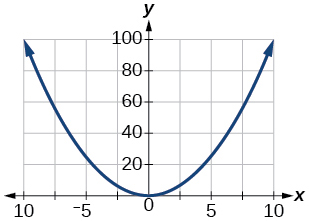$\left[-100,100\right]$

For the following exercises, graph $\text{\hspace{0.17em}}y={x}^{3}\text{\hspace{0.17em}}$ on the given viewing window. Determine the corresponding range for each viewing window. Show each graph.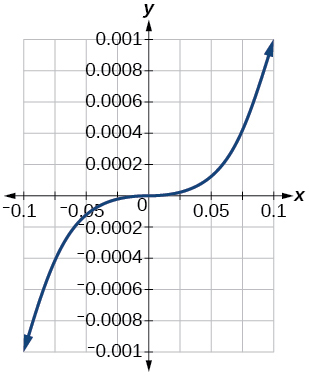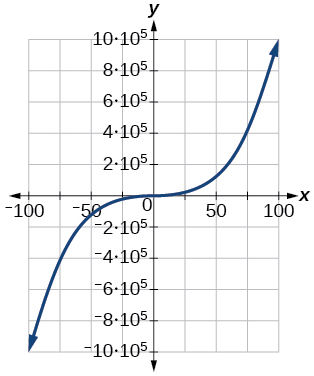For the following exercises, graph $\text{\hspace{0.17em}}y=\sqrt{x}\text{\hspace{0.17em}}$ on the given viewing window. Determine the corresponding range for each viewing window. Show each graph.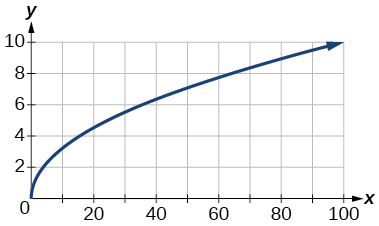For the following exercises, graph $y=\sqrt{x}$ on the given viewing window. Determine the corresponding range for each viewing window. Show each graph.

$\left[-0.001,\text{0.001}\right]$

$\left[-0.1,\text{0.1}\right]$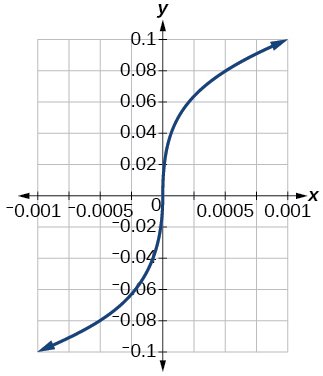$\left[-1000,\text{1000}\right]$

$\left[-1,000,000,\text{1,000,000}\right]$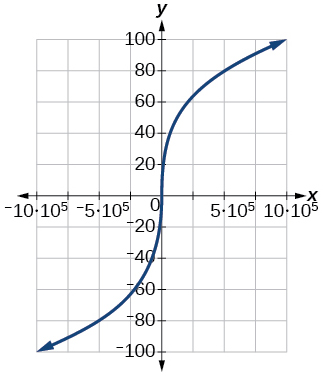## Real-world applications

The amount of garbage, $\text{\hspace{0.17em}}G,\text{\hspace{0.17em}}$ produced by a city with population $\text{\hspace{0.17em}}p\text{\hspace{0.17em}}$ is given by $\text{\hspace{0.17em}}G=f\left(p\right).\text{\hspace{0.17em}}$ $G\text{\hspace{0.17em}}$ is measured in tons per week, and $\text{\hspace{0.17em}}p\text{\hspace{0.17em}}$ is measured in thousands of people.

1. The town of Tola has a population of 40,000 and produces 13 tons of garbage each week. Express this information in terms of the function $\text{\hspace{0.17em}}f.\text{\hspace{0.17em}}$
2. Explain the meaning of the statement $\text{\hspace{0.17em}}f\left(5\right)=2.$

The number of cubic yards of dirt, $\text{\hspace{0.17em}}D,\text{\hspace{0.17em}}$ needed to cover a garden with area $\text{\hspace{0.17em}}a\text{\hspace{0.17em}}$ square feet is given by $\text{\hspace{0.17em}}D=g\left(a\right).$

1. A garden with area 5000 ft 2 requires 50 yd 3 of dirt. Express this information in terms of the function $\text{\hspace{0.17em}}g.$
2. Explain the meaning of the statement $\text{\hspace{0.17em}}g\left(100\right)=1.$

a. $\text{\hspace{0.17em}}g\left(5000\right)=50;$ b. The number of cubic yards of dirt required for a garden of 100 square feet is 1.

Let $\text{\hspace{0.17em}}f\left(t\right)\text{\hspace{0.17em}}$ be the number of ducks in a lake $\text{\hspace{0.17em}}t\text{\hspace{0.17em}}$ years after 1990. Explain the meaning of each statement:

1. $f\left(5\right)=30$
2. $f\left(10\right)=40$

Let $\text{\hspace{0.17em}}h\left(t\right)\text{\hspace{0.17em}}$ be the height above ground, in feet, of a rocket $\text{\hspace{0.17em}}t\text{\hspace{0.17em}}$ seconds after launching. Explain the meaning of each statement:

1. $h\left(1\right)=200$
2. $h\left(2\right)=350$

a. The height of a rocket above ground after 1 second is 200 ft. b. the height of a rocket above ground after 2 seconds is 350 ft.

Show that the function $\text{\hspace{0.17em}}f\left(x\right)=3{\left(x-5\right)}^{2}+7\text{\hspace{0.17em}}$ is not one-to-one.

what is set?
a colony of bacteria is growing exponentially doubling in size every 100 minutes. how much minutes will it take for the colony of bacteria to triple in size
I got 300 minutes. is it right?
Patience
no. should be about 150 minutes.
Jason
It should be 158.5 minutes.
Mr
ok, thanks
Patience
100•3=300 300=50•2^x 6=2^x x=log_2(6) =2.5849625 so, 300=50•2^2.5849625 and, so, the # of bacteria will double every (100•2.5849625) = 258.49625 minutes
Thomas
what is the importance knowing the graph of circular functions?
can get some help basic precalculus
What do you need help with?
Andrew
how to convert general to standard form with not perfect trinomial
can get some help inverse function
ismail
Rectangle coordinate
how to find for x
it depends on the equation
Robert
yeah, it does. why do we attempt to gain all of them one side or the other?
Melissa
whats a domain
The domain of a function is the set of all input on which the function is defined. For example all real numbers are the Domain of any Polynomial function.
Spiro
Spiro; thanks for putting it out there like that, 😁
Melissa
foci (–7,–17) and (–7,17), the absolute value of the differenceof the distances of any point from the foci is 24.
difference between calculus and pre calculus?
give me an example of a problem so that I can practice answering
x³+y³+z³=42
Robert
dont forget the cube in each variable ;)
Robert
of she solves that, well ... then she has a lot of computational force under her command ....
Walter
what is a function?
I want to learn about the law of exponent
explain this
what is functions?
A mathematical relation such that every input has only one out.
Spiro
yes..it is a relationo of orders pairs of sets one or more input that leads to a exactly one output.
Mubita
Is a rule that assigns to each element X in a set A exactly one element, called F(x), in a set B.
RichieRich

#### Get Jobilize Job Search Mobile App in your pocket Now!ByByBy John GabrieliBy OpenStaxBy Rohini AjayBy Dakota BocanBy JavaChamp TeamBy OpenStaxBy OpenStaxBy DanielrosenbergerBy Stephen VoronBy OpenStax